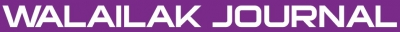### A New (G'/G)-Expansion Method and Its Application to the Burgers Equation

Md. Nur ALAM, M. Ali AKBAR

#### Abstract

In this article, a new (G'/G)-expansion method is proposed, where G = G(S) satisfies a second order nonlinear ordinary differential equation to seek the travelling wave solutions of nonlinear evolution equations. The Burgers equation is chosen to illustrate the validity and advantages of proposed method. Hyperbolic function, trigonometric function and rational function solutions with arbitrary constants are obtained from which some special solutions, including the known solitary wave solution, are derived by setting the appropriate values of constants. It is shown that the new (G'/G)-expansion method is effective, and gives new, more general, travelling wave solutions than the existing methods, such as the basic (G'/G)-expansion method, the extended (G'/G)-expansion method, the improved (G'/G)-expansion method, the generalized and improved (G'/G)-expansion method etc.

doi:10.14456/WJST.2014.86

#### Keywords

(G'/G)-expansion method, The Burgers equation, Nonlinear differential equation, Homogeneous balance, Traveling wave solutions, Solitary wave solutions

PDF

#### References

MJ Ablowitz and PA Clarkson. Soliton, Nonlinear Evolution Equations and Inverse Scattering. Cambridge University Press, New York, 1991.

VB Matveev and MA Salle. Darboux Transformation and Solitons. Springer, Berlin, 1991.

MR Miura. Backlund Transformation. Springer, Berlin, 1978.

R Hirota. The Direct Method in Soliton Theory. Cambridge University Press, Cambridge, 2004.

W Malfliet and W Hereman. The tanh method: II. Perturbation technique for conservative systems. Phys. Scr. 1996; 54, 563-9.

GW Bluman and S Kumei. Symmetries and Differential Equations. Springer-Verlag, Berlin, 1989.

J Weiss, M Tabor and G Carnevale. The Painlevé property for partial differential equations. J. Math. Phys. 1983; 24, 522-6.

JH He and XH Wu. Exp-function method for nonlinear wave equations. Chaos Soliton. Fract. 2006; 30, 700-8.

ST Mohyud-Din, MA Noor and KI Noor. Exp-function method for solving higher-order boundary value problems. Bull. Institute Math. 2009; 4, 219-34.

A Yildirim and Z Pinar. Application of the exp-function method for solving nonlinear reaction-diffusion equations arising in mathematical biology. Comput. Math. Appl. 2010; 60, 1873-80.

E Misirli and Y Gurefe. Exp-function method for solving nonlinear evolution equations. Math. Comput. Appl. 2011; 16, 258-66.

W. Zhang. The extended tanh method and the exp-function method to solve a kind of nonlinear heat equation. Math. Probl. Eng. 2010; 2010, Article ID 935873, 12 pages.

M Wang, X Li and J Zhang. The -expansion method and traveling wave solutions of nonlinear evolution equations in mathematical physics. Phys. Lett. A 2008; 372, 417-23.

MA Akbar, NHM Ali and EME Zayed. Abundant exact traveling wave solutions of the generalized Bretherton equation via -expansion method. Comm. Theor. Phys. 2012; 57, 173-8.

N Taghizade1 and A Neirameh. The solutions of TRLW and Gardner equations by -expansion method. Int. J. Nonlinear Sci. 2010; 9, 305-10.

H. Zhang. New application of the -expansion method. Commun. Nonlinear Sci. Numer. Simulat. 2009; 14, 3220-25.

GR Kol1 and CB Tabi. Application of the -expansion method to nonlinear blood flow in large vessels. Phys. Scr. 2011; 83, 045803.

MA Akbar, NHM Ali and EME Zayed. A generalized and improved -expansion method for nonlinear evolution equations. Math. Probl. Eng. 2012; 2012, Article ID 459879.

EME Zayed and KA Gepreel. Some applications of the -expansion method to non-linear partial differential equations. Appl. Math. Comput. 2009; 212, 1-13.

A Bekir. Application of the -expansion method for nonlinear evolution equations. Phys. Lett. A 2008; 372, 3400-6.

EME Zayed. The -expansion method and its applications to some nonlinear evolution equations in the mathematical physics. J. Appl. Math. Comput. 2009; 30, 89-103.

S Zhang, J Tong and W Wang. A generalized -expansion method for the mKdV equation with variable coefficients. Phys. Lett. A 2008; 372, 2254-7.

J Zhang, X Wei and Y Lu. A generalized -expansion method and its applications. Phys. Lett. A 2008; 372, 3653-8.

J Zhang, F Jiang and X Zhao. An improved -expansion method for solving nonlinear evolution equations. Int. J. Comput. Math. 2010; 87, 1716-25.

EME Zayed. New traveling wave solutions for higher dimensional nonlinear evolution equations using a generalized -expansion method. J. Phys. A Math. Theor. 2009; 42, 195202-14.

EME Zayed. The -expansion method combined with the Riccati equation for finding exact solutions of nonlinear PDEs. J. Appl. Math. Inform. 2011; 29, 351-67.

H Kheiri, MR Moghaddam and V Vafaei. Application of the -expansion method for the Burgers, Burgers-Huxley and modified Burgers-KdV equations. Pramana. J. Phys. 2011; 76, 831-42.

### Refbacks

• There are currently no refbacks.Online ISSN: 2228-835X

http://wjst.wu.ac.th

Last updated: 20 June 2019